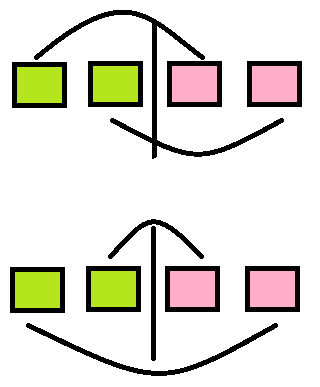## Solution:

Since you can do any number of operations, in order to simplify the problem, we can specify $x$ as 1.

First of all, it's very clear that: if $a_i>avg(a)$, then $a_i$ will be the source; if $a_i<avg(a)$, then it will be the sink; if $a_i=avg(a)$ , Then will no do anything on it.

Considering why the maximum and minimum values are the same, we can observe the following picture:Red is the value above the average, green is the value below the average

For any operation, according to the above picture, swapping the order will not cause a cost change. That's the reason the maximum and minimum cost is the same.

Then there is a special case when there is only one red or green part. This situation can be arranged no matter how it is arranged.

Finally, it should be noted that if the number appears multiple times, then all permutations of these numbers must be removed.

## Code:蜀ICP备19018968号